# The Inertia Tensor .... Determining Components of Angular Momentum ....

• I
• Math Amateur

#### Math Amateur

Gold Member
MHB
TL;DR Summary
I am having trouble following Neuenschwander's logic in deriving the components of angular momentum ... as he proceed to derive the components of the inertia tensor
I am reading Tensor Calculus for Physics by Dwight E. Neuenschwander and am having difficulties in following his logic regarding proceeding to derive the components of Angular Momentum and from there the components of the Inertia Tensor ...

On page 36 we read the following: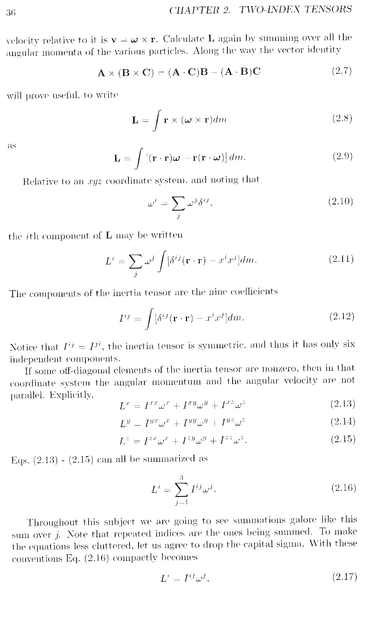In the above text by Neuenschwander we read the following:

" ... ... ## L = \int [ ( r \bullet r ) \omega - r ( r \bullet \omega ) ] dm ## ... ... ... (2.9)

Relative to an xyz coordinate system and noting that

## \omega^i = \sum_j \omega^j \delta^{ij} ## ... ... ... (2.10)

the ith component of L may be written

## L^i =\sum_j \omega^j \ \int [ \delta^{ij} ( r \bullet r ) - x^i x^j] dm ## ... ... ... (2.11) ... ... ... "

Can some please (... preferably in some detail) show me how Neuenschwander derives the expression for ## L^i ### (that is equation 2.11 from the equations above 2.11 ...

Help will be much appreciated ...

Now I think it will be helpful for readers of the above post to have access to the start of Section 2.2 so i am providing this ... as follows: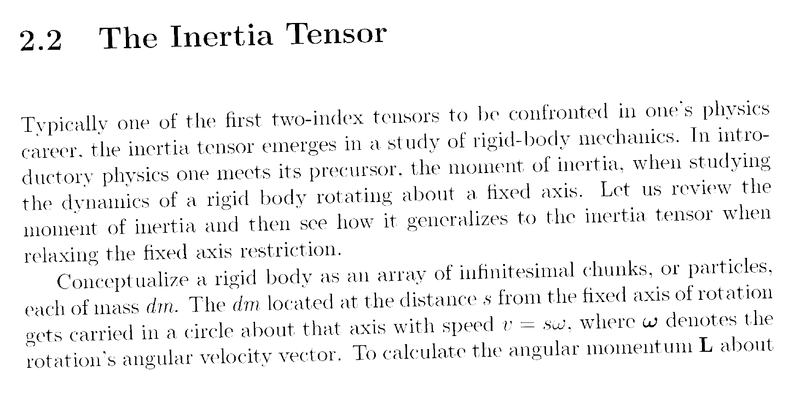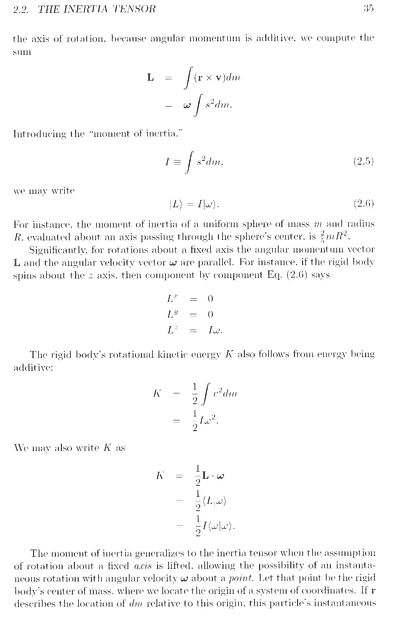'
Hope that helps

Peter

Last edited:
There is not much to be shown as it is just writing equation 2.9 on index form while keeping 2.10 in mind.

Thanks for the reply Orodruin ... Given that it should be straight forward i had another shot at demonstrating the Neuenschwander Equation 2.9 leads to (the compact and rather non-intuitive IMO) equation 2.11 ... I think my demonstration is OK ... but not completely sure ... details follow ...

Peter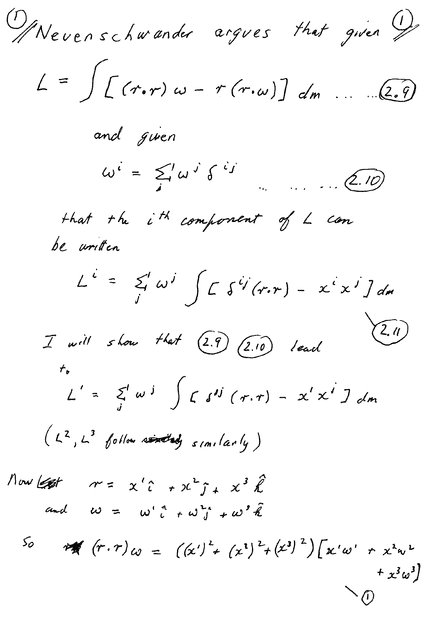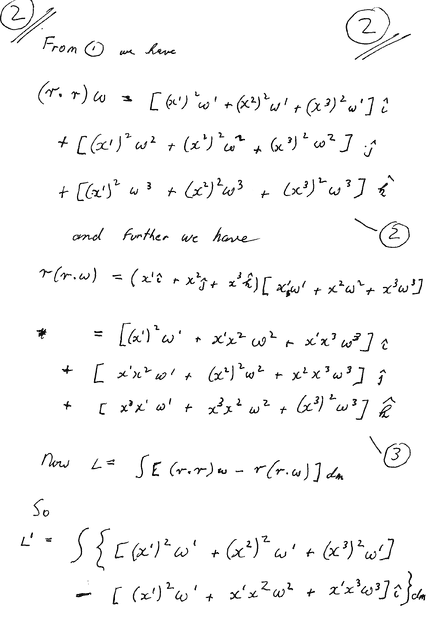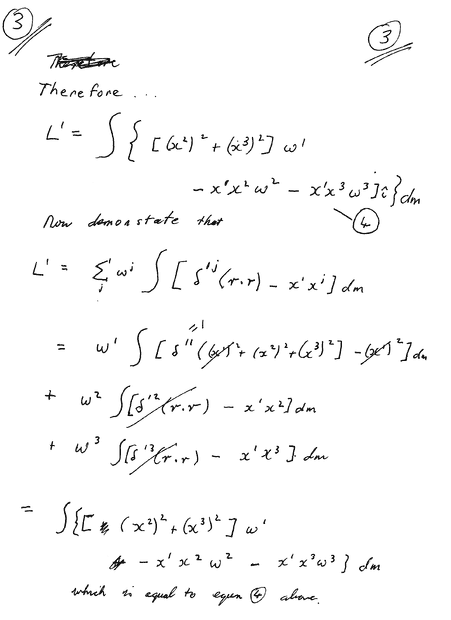Hope that is OK ...

Peter

You are way overcomplicating things by writing out all the terms explicitly. The ith component of any vector ##\vec v## is given by ##v_i = \vec e_i \cdot \vec v## and therefore
$$L_i = \vec e_i\cdot \vec L = \int [r^2 \vec e_i\cdot\vec\omega - (\vec e_i\cdot \vec x)(\vec x\cdot \vec \omega)] dm = \int [r^2 \omega_i - x_i (\vec x\cdot \vec \omega)] dm.$$
Now use that ##\omega_i = \delta_{ij}\omega_j## and ##\vec x \cdot \vec \omega = x_j \omega_j## (implicit sums over j due to the summation convention) and you obtain
$$L_i = \int [r^2 \delta_{ij}- x_i x_j]\omega_j dm$$
where ##\omega_j## is constant and can be moved out of the integral.

Last edited:
•Math Amateur
Thanks Orodruin … most helpful …